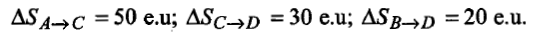Change in enthalpy for reaction,

2H2O2(l)$\to$ 2H2O(l) + O2(g)

if the heat of formation of H2O2(l) and H2O(l) are -188 and -286 kJ/mol respectively is

(a) -196 kJ/mol              (b) + 196 kJ/mol

(c) +948 kJ/mol             (d) -948 kJ/mol

Concept Questions :-

Enthalpy and It's Type
High Yielding Test Series + Question Bank - NEET 2020

Difficulty Level:

One mole of a non-ideal gas undergoes a change of state (2.0 atm, 3.0 L, 95 K)$\to$(4.0 atm, 5.0 L, 245 K) with a change in internal energy, $∆$U = 30.0 L atm. The change in enthalpy ($∆$H) of the process in L atm is:

(a) 40.0

(b) 42.3

(c) 44.0

(d) not defined, because pressure is not constant

Concept Questions :-

First Law of Thermodynamics
High Yielding Test Series + Question Bank - NEET 2020

Difficulty Level:

At absolute zero, the entropy of a perfect crystal is zero. This is........... of thermodynamics.

(a) first law               (b) second law

(c) third law              (d) none of these

Concept Questions :-

Spontaneity and Entropy
High Yielding Test Series + Question Bank - NEET 2020

Difficulty Level:

At constant pressure and temperature, the direction of any chemical reaction is one where, the...... decrease.

(a) entropy                (b) enthalpy

(c) Gibbs energy        (d) none of these

Concept Questions :-

Gibbs Energy Change
High Yielding Test Series + Question Bank - NEET 2020

Difficulty Level:

The work done by a mass less piston in causing an expansion $∆$V (at constant temperature), when the opposing pressure, P is variable, is given by:

(a) W= $-\int \mathrm{P}∆\mathrm{V}$                (b) W=0

(c) W= -P$∆$V                      (d) none of these

Concept Questions :-

Internal Energy and work done
High Yielding Test Series + Question Bank - NEET 2020

Difficulty Level:

Entropy decreases during:

(a) crystallization of sucrose from solution

(b) rusting of iron

(c) melting of ice

(d) vaporization of camphor

High Yielding Test Series + Question Bank - NEET 2020

Difficulty Level:

Entropy change of vaporisation at constant pressure is given by:

Concept Questions :-

Spontaneity and Entropy
High Yielding Test Series + Question Bank - NEET 2020

Difficulty Level:

What is the entropy change for the reaction given below,

2H2 (g) + O2 (g) $\to$2H2O(l)

at temperature 300 K? Standard entropies of H2 (g), O2(g) and H2O(l) are 126.6, 201.20 and 68.0 JK-1mol-1 respectively.

(a) -318.4 JK-1mol-1                           (b) 318.4 JK-1mol-1

(c) 31.84 JK-1mol-1                             (d) none of these

Concept Questions :-

Spontaneity and Entropy
High Yielding Test Series + Question Bank - NEET 2020

Difficulty Level:

If S$°$ for H2, Cl2 and HCl are 0.13, 0.22 and 0.19 kJ K-1mol-1 respectively. The total change in standard entropy for the reaction, H2 + Cl2 $\to$2HCl is:

(a) 30 J K-1mol-1                         (b) 40 J K-1mol-1

(c) 60 J K-1mol-1                         (d) 20 J K-1mol-1

Concept Questions :-

Spontaneity and Entropy
High Yielding Test Series + Question Bank - NEET 2020

Difficulty Level:

The direct conversion of A to B is difficult and thus it is converted by path A$\to$C$\to$D$\to$B. GivenWhere e.u. is the entropy unit, then $∆{\mathrm{S}}_{\mathrm{A}\to \mathrm{B}}$ is:

(a) +60 e.u                                       (b) +100 e.u

(c) -60 e.u                                        (d) -100 e.u

Concept Questions :-

Hess's Law